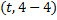# Absolute value of the sum of the abscissas of the points on the linethat lie at a unit distance from the lineis

## Question ID - 55433 :- Absolute value of the sum of the abscissas of the points on the linethat lie at a unit distance from the lineis

3537

(4)

Any point on the lineiswhereNow distance of this point from the lineis1orsum of values isNext Question :

The number of values offor which the linesandare coincident